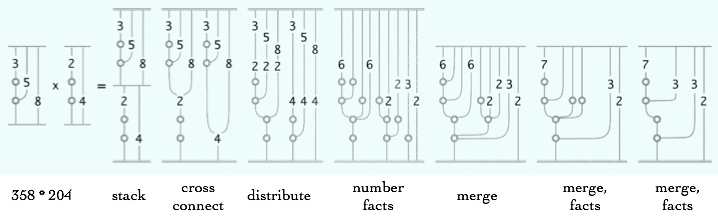# Network Numbers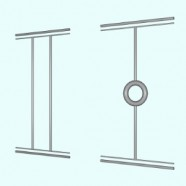Iconic numbers can be expressed as networks suitable for a fine-grain silicon implementation.  Network numbers have a top and a bottom.  Addition is connecting top-to-top and bottom-to-bottom.  Multiplication is connecting bottom-to-top.  The Image shows the binary simplification rule for these numbers.  Two wires, which represent two units, become one wire with a loop.  The loop stands in place of a doubler (shift-right in a hardware register). Network numbers follow the same rules as unit ensemble arithmetic with binary depth-value.  The binary iconic calculator follows the same evaluation sequence but with numbers expressed in the container variety of iconic arithmetic.  Decimal networks are illustrated at the end of the page. The following content is updated from a 1987 internal whitepaper I wrote at Advanced Decision Systems.

Network numbers are a formal redefinition of the concept of number.  Rather than being inert objects that are operated upon, network numbers are active objects that compute themselves.  This is a fundamental refocusing of the concepts of object and operator.  Rather than building arithmetic from easily stated and relatively useless numerical objects coupled with computationally intensive operators, network numbers are active objects that dynamically compute their value coupled with easily stated and relatively inert operators.  Adding and multiplying network numbers is a trivial process.  Addition is independent of the magnitude of the numbers being added; multiplication requires at most log n effort, where n is the magnitude of the smallest number being multiplied.

The computational effort in using network numbers is in determining the value of a network number rather than in adding or multiplying them.  Reading a network number is strongly parallel and is more efficient than traditional computation.  Reading a value is log(n), where n is the number of bits in the binary representation of the result.  And reading is required only once, when the result of any combination of operations is desired.

This video shows that the binary number 14 can be constructed in one, two and three dimensions.  The one-dimensional textual version dominates our concept of number, and thus limits our understanding of the broader nature of numbers.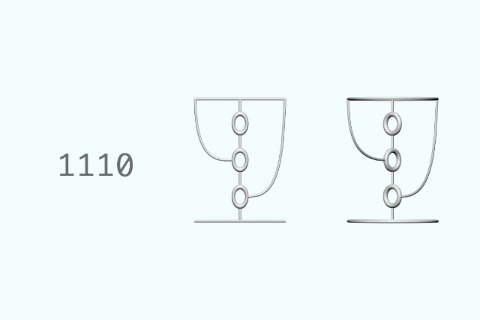Binary 14 in one, two and three dimensions

## Definitions

A boundary number has a top and a bottom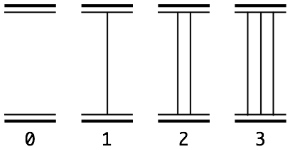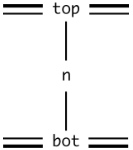We will call the bottom of a network number the bot.  The magnitude of the number, n, is expressed by the connectivity between the top and the bot. The network equivalent of a unit ensemble number is a collection of single wires connecting bot to top. Zero has no representation, it is the absence of a connection. One has simple wire connectivity. Multiple units are represented by multiple wires.

The network top and bot bound a parallel computational space. The wires form a network of connectivity that represents the value of a number. This network can be converted into a standard form that can be recognized as a conventional binary value by the network top.

## Standardization

The parallel standardization process is rather like compiling the network representation.  Standardization is desirable for input and output, and for resource management during transformation.  Standardization minimizes the effort of reading a network number.  Here are the standardized network numbers from 1 to 16 in base-2: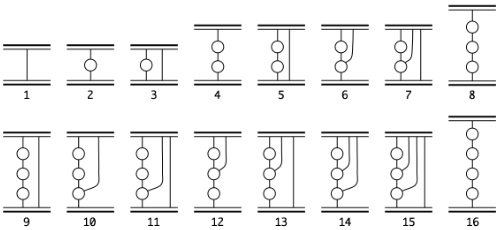The standardization process uses the same two operations implemented in the binary iconic calculator, Group and Merge.  The Group operation transforms a pair of simple connections into a binary multiplier represented by a loop. The Merge operation combines loops that share the same bot.  Merging generalizes to any number of rings at the same time, and it is independent of the base. In the textual notation of depth-value unit ensembles, the two standardization operations are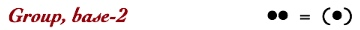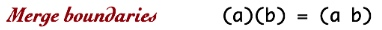In the notation of network numbers, these rules are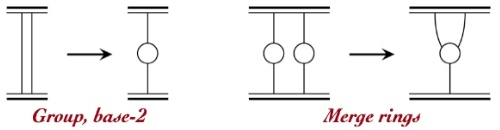Both Group and Merge apply to any level in the boundary number, not just the top and bot, and all operations can occur in parallel.

Here is an animation showing the simple binary group operation.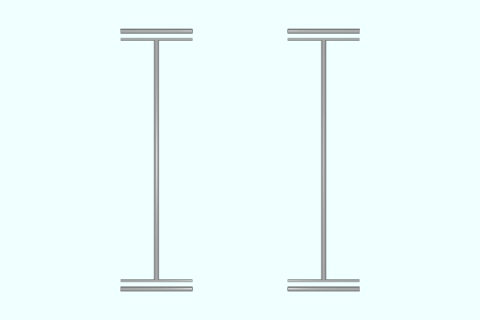Group two wires

Here is an animation showing the simple merge operation.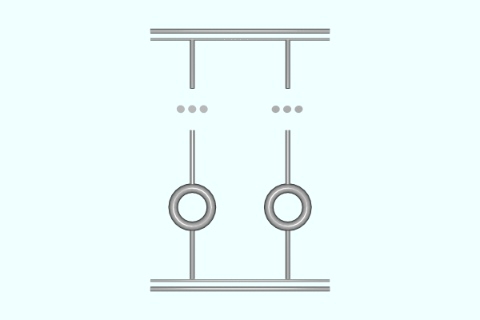Merge any number of rings

In the following animation the two operations are put together to standardize four unit wires.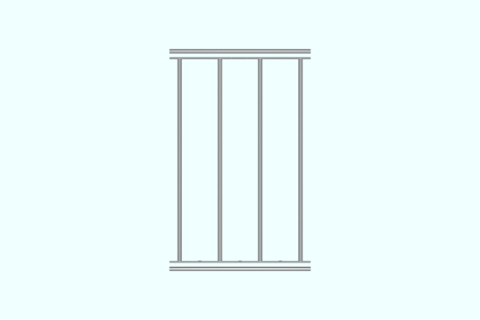Standardize four wires

## Multiple Representations

The same network number has many different representations, that is, many different networks of connectivity.  The example shows several different networks for the number 18.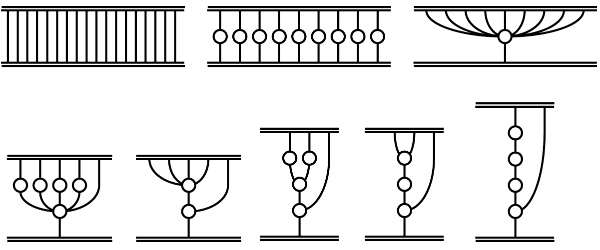A network number reader would return the same conventional number for each representation.   There is only one canonical minimal form, the lower right form above.  Successive application of Group and Merge will produce the minimal form.  Standardization rules can be applied in parallel since every node is top to its lower neighbors and every node is bot to its upper neighbors.  The above eight representations of 18, from left to right,  also show the reduction sequence from the unit ensemble at the top left to the standardized minimal network at the bottom left.  Written in the parens/dot notation, this sequence is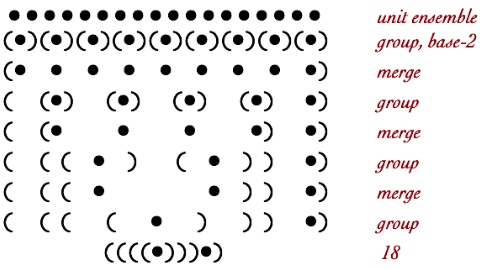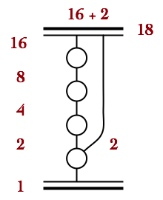To read a network number, start at the bot (the most deeply nested unit).  Passing upward through a loop (crossing a boundary outward) doubles the value of the current upward tally (i.e. the lower network).  When two subnetworks branch out from a common loop, their values add when the subnetworks reach the top.  For example, to read the network form of the number 18, begin with 1 at the bottom.  Passing through the first ring yields 2.  At this point a second path diverges, carrying the value 2 to the top.  The original path carrying 2 continues upward, passing through three more doublers (4, 8, 16), finally sending the value 16 to the top.  At the top, 16 is added to 2 to yield 18.

The process is the same for base-10, except that the grouping operation collects ten units to form one ring.

## Numerical Operations

In iconic arithmetic, the operations of addition and multiplication are achieved simply by putting numbers together.  For network numbers, putting together side-by-side achieves addition, while putting together bot-to-top achieves multiplication.  In computational terms, changing two pointers is sufficient to perform each elementary operation.

Addition is implemented by merging tops with tops and bots with bots.  Any number of additions can occur at the same time.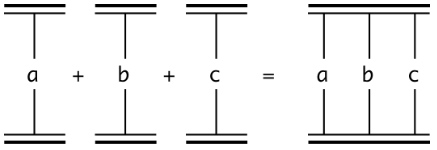Here is an animation showing 7 added to 5.  The network numbers are joined together and then the Group and Merge operations standardize the network form.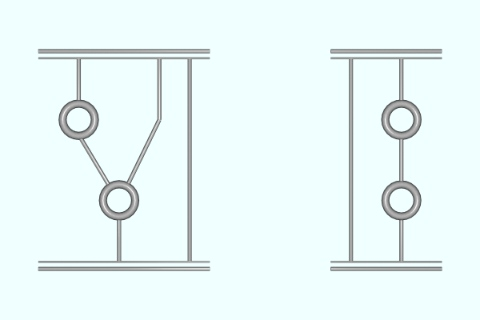Negative numbers are defined by reversing top and bot.  When tops and bots are connected, networks then have a gradient.  Networks flowing upward (bot to top) are positive, networks flowing downward (top to bot) are negative. Subtraction is then the addition of networks with gradients.  One additional standardization rule is necessary, the network analogy of the unit Cancel rule.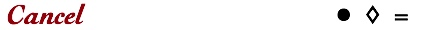Characteristic of iconic notation, the canceling pair disappears completely.  The network notation for the Cancel transformation is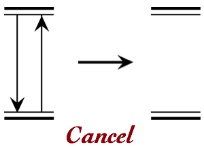### Multiplication

Multiplication is implemented by joining tops with bots. This is achieved simply by stacking the network numbers.  Removing the combined top/bot bar achieves multiplication of the upper number by the lower number.  Stacking is a form of substitution, for which the outputs of the top bar for the lower number gets connected to the inputs of the bot bar for the upper number.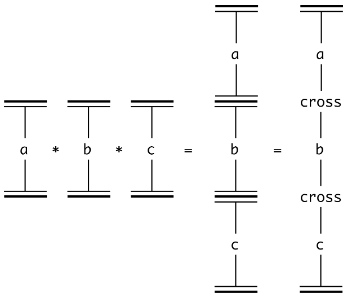When there is more than one wire attached to the top or bot bar, the multiplied networks must be cross-connected. To cross-connect, connect all top wires of the lower network to all bot wires of the upper network. The complete cross-connection of one network with another is a physical model of the Multiplicative Principle, that each unit/wire in one group is converted into an entire other group.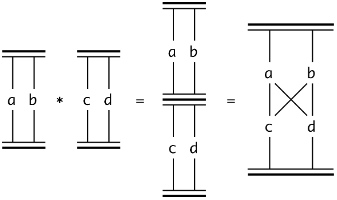As an example, the following two animations show 7*5 and 5*7.  In both animations, the networks numbers are first stacked, then cross-connected.  The connections are distributed and then Group and Merge standardize the result.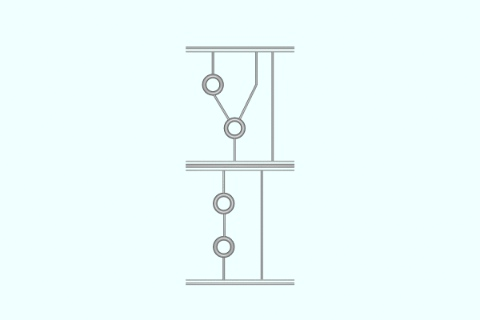Multiply 7 by 5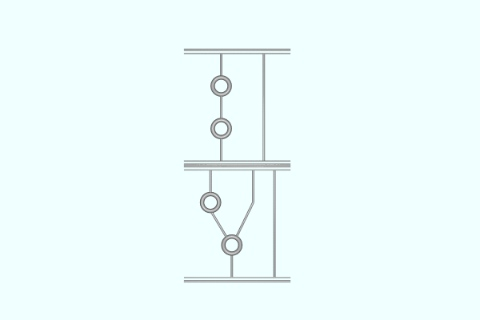Multiply 5 by 7

## Algebraic Rules

Reading a network number involves traversing all available paths. The cross-connected wiring generated by multiplication is in factored form. By separating the multiple connections, the factored form is converted into the polynomial form of the product. Cross-connection is thus a succinct embodiment of what must be done to multiply polynomials (of which numbers area subcase).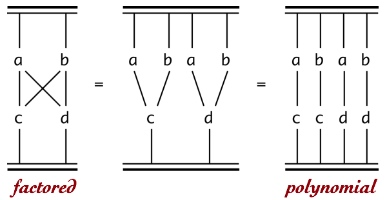In conventional notation,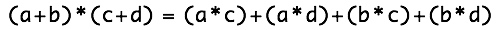Obviously the commutativity, associativity and identity rules of conventional algebra are not relevant to the network model. The inverse rule for addition is expressed by a network gradient. The distributive rule for network numbers is the Merge operation.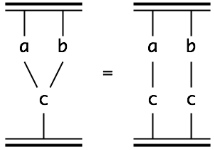which would be written in symbolic form as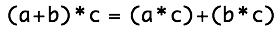The variables in the algebraic networks stand in place of any network of connectivity between top and bot. That is, any network can be inserted in place of a network variable, just like any symbolic expression can be inserted in place of a symbolic variable.

In the network model, a form at any level can propagate up the network, exchanging positions with other forms. Whenever the moving form encounters a branch, to continue to propagate upwards, the form must be replicated once for each upward path. This is another form of network distribution.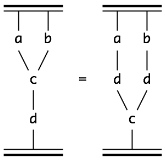### Examples

Network number notation provides an elegant representation of the binomial theorem (i.e. the powers of sums):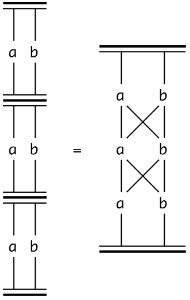The right-hand-side represents eight paths from bot to top. One path passes through a three times; three paths pass through a twice and b once. Similarly, three paths pass through b twice and a once. One path passes through b three times. In conventional notation,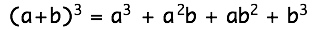Finally, abutting and stacking network numbers provides a new perspective on the Sum and Product (i.e. factorial) operations.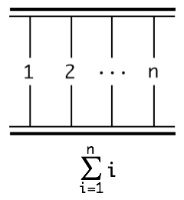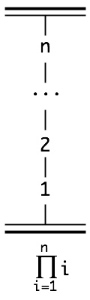## Decimal Networks

Although the network model was not designed for decimal computation, decimal networks can be constructed using the same principles as binary networks. The primary difference is that rings group ten unit connections. There is also a pragmatic difference. Grouping must be extended to include the sums of digits (commonly called the addition tables). The examples below show addition and multiplication of decimal networks.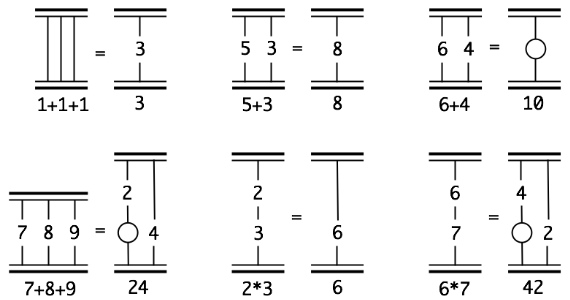Here is a larger addition, 358+204. The steps that achieve addition are spread out left-to-right. First 358 and 204 are put together. This result is then standardized by grouping digits while applying addition number facts to from groups, and merging rings as they occur.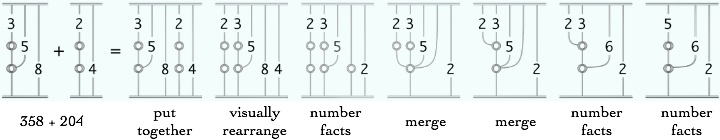Next is a larger multiplication. The first figure shows 204*358, while the second shows 358*204. For the multiplication, first the two decimal networks are stacked and cross-connected. This result is then standardized by grouping digits via multiplication digit facts and merging rings.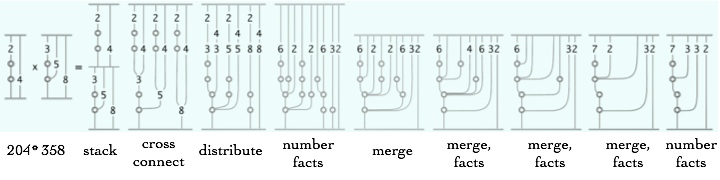The next figure shows 358*204. For the multiplication, first the two decimal networks are stacked and cross-connected. This result is then standardized by applying multiplication digit facts and merging rings.